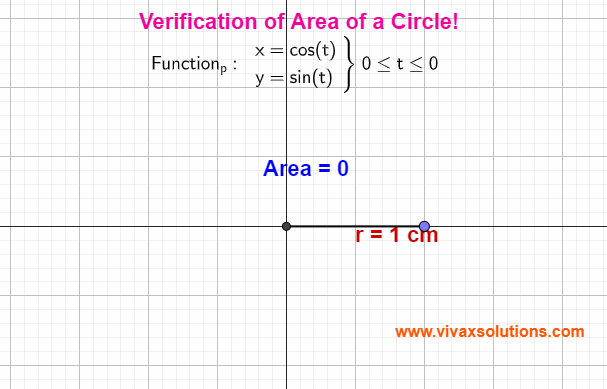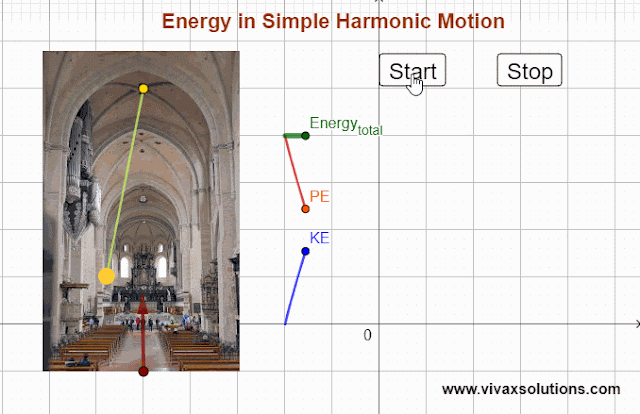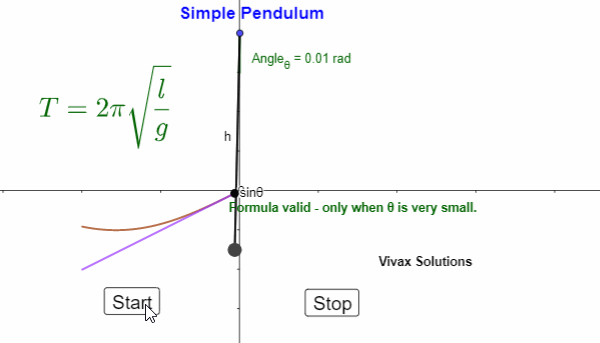## Posts

Showing posts from November 20, 2022

### Verification of the area of a circleArea of a circle by parametric equations The proof with the aid of parametric equation is as follows:

### Energy changes in simple harmonic motionThe above simulation of the simple harmonic motion involving a pendulum shows how the potential energy, PE and kinetic energy, KE, change with the position of the pendulum, provided that the latter swings through a small angle. As you can see the total energy of the system remains the same: the loss of KE turns into PE and vice versa. In order to practise this interactively, along with a few related simulations under the SHM, please follow the link below: A comprehensive and interactive tutorial on the SHM is here .

### Simple Harmonic Motion of PendulumThe above simulation shows how the angle of oscillation determines the validity of the formula of the simple pendulum. During the process of deriving the formula, we assume the angle is small and measured in radians.  For the full tutorial, please follow this link .